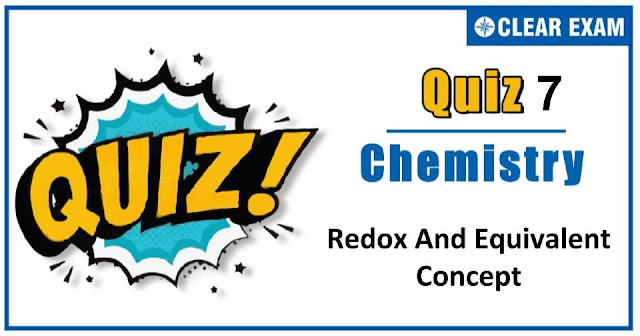Redox And Equivalent Concept Quiz-7

The one subject in NEET which is candidates who can easily attain good marks is Chemistry. That's the reason, often, one doesn’t pay notice and choose to compromise it. But if one wants to rank above others, the tip is to be thorough with NEET chemistry concepts. The understanding of reactions and definite basic understanding is what requires major attention in Chemistry but once done it only gets simpler from there. The main focus on the to-do list should be on getting a hang of the NCERT syllabus of NEET chemistry..

Q1. The compound that can work both as an oxidising and reducing agent is:
•  KMnO4
•  H2 O2
•  Fe2 (SO4 )3
•  K2 Cr2 O7
Solution
(b) Oxygen in H2 O2 has ox.no. −1 which can increase or decrease.

Q2.A group of methods of quantitative chemical analysis involving the measurement of volume of reacting substance is known as :
•  Gravimetric analysis
•  Volumetric analysis
•  Both (a) and (b)
•  None of the above
Solution
(b) It is definition of volumetric analysis.

Q3. When K2 Cr2 O7 is converted into K2 CrO4, the change in oxidation number of chromium is
•  0
•  5
•  7
•  9
Solution
(a) Let oxidation state of Cr in K2 Cr2 O7=x (+1×2)+2x+(-2×7)=0
or +2+2x-14=0
∴ x=+6 Let oxidation state of Cr in K2 CrO4=x +1×2+x+(-2×4)=0 2+x-8=0 x=6
∴ Change in oxidation state of Cr is zero when it changes from K2 Cr2 O7 to K2 CrO4.

Q4. NO3- ions are converted to NH4+ ions by a suitable reactant. The equivalent mass of NO3- and NH4+ are :
•  7.75, 2.25
•  7.75, 7.75
•  2.25, 7.75
•  2.25, 2.25

Q5. During a redox titration involving a solution containing Fe2+ ions against MnO4- in the presence of excess of H+ ions, the number of electrons that gets transferred is
•  6
•  5
•  4
•  2

Q6. In the reaction, SO2+2H2 S⟶3S+2H2 O, the substance oxidised is
•  H2 S
•  SO2
•  S
•  H2 O

Q7.As the oxidation state for any metal increases, the tendency to show ionic nature:
•  Decreases
•  Increases
•  Remains same
•  None of these
Solution
(a) Tendency to lose more electron for cation decreases.

Q8.Addition of zinc powder to CuSO4 solution precipitates copper due to :
•  Reduction of Cu2+
•  Reduction of SO42-
•  Reduction of Zn
•  Hydrolysis of CuSO4
Solution
(a) Cu2++2e ⟶Cu

Q9.Oxidation number of Fe in K3 [Fe(CN)6] is :
•  +2
•  +3
•  +4
•  +1
Solution
(b) 3 × 1+a+6 × (-1)=0 ∴a= +3

Q10. Oxidation number of S in S2 O32-is :
•  +2
•  -2
•  4
•  Zero
Solution
(a) 2 × a+3 × (-2)= -2 ∴a= +2Written by: AUTHORNAME

AUTHORDESCRIPTIONWant to know more

Please fill in the details below:

Latest NEET Articles\$type=three\$c=3\$author=hide\$comment=hide\$rm=hide\$date=hide\$snippet=hide

Name

ltr
item
BEST NEET COACHING CENTER | BEST IIT JEE COACHING INSTITUTE | BEST NEET, IIT JEE COACHING INSTITUTE: redox-and-equivalent-concept-quiz-7
redox-and-equivalent-concept-quiz-7